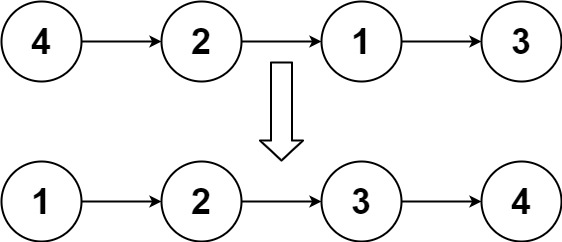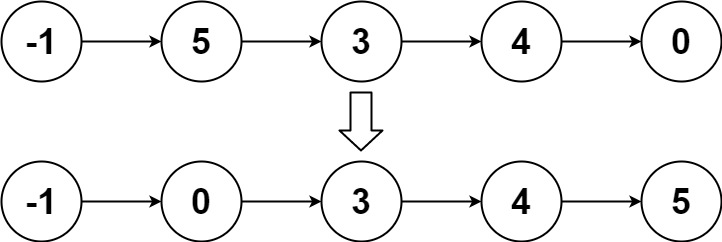148. Sort List
Medium
9.5K
285

Given the `head` of a linked list, return the list after sorting it in ascending order.

Example 1:```Input: head = [4,2,1,3]
Output: [1,2,3,4]
```

Example 2:```Input: head = [-1,5,3,4,0]
Output: [-1,0,3,4,5]
```

Example 3:

```Input: head = []
Output: []
```

Constraints:

• The number of nodes in the list is in the range `[0, 5 * 104]`.
• `-105 <= Node.val <= 105`

Follow up: Can you sort the linked list in `O(n logn)` time and `O(1)` memory (i.e. constant space)?

Accepted
616.6K
Submissions
1.1M
Acceptance Rate
55.1%

Seen this question in a real interview before?
1/4
Yes
No

Discussion (0)

Related Topics